# Intermediate Geometry : How to find the length of an arc

## Example Questions

1 3 Next →

### Example Question #91 : Intermediate Geometry

Find the length of an arc if the radius of the circle isand the measurement of the central angle isdegrees.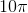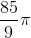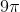Explanation:

An arc is just a piece—or a fraction—of a circle's circumference. Use the following formula to find the length of an arc: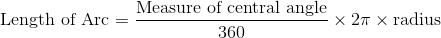Substitute in the given values for the central angle and the radius.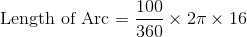Solve.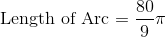### Example Question #92 : Intermediate Geometry

Find the length of an arc if the radius of the circle isand the measurement of the central angle isdegrees.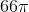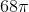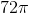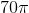Explanation:

An arc is just a piece—or a fraction—of a circle's circumference. Use the following formula to find the length of an arc:Substitute in the given values for the central angle and the radius.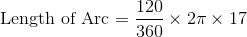Solve.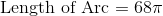### Example Question #93 : Intermediate Geometry

Find the length of an arc if the radius of the circle isand the measurement of the central angle isdegrees.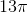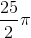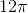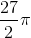Explanation:

An arc is just a piece—or a fraction—of a circle's circumference. Use the following formula to find the length of an arc:Substitute in the given values for the central angle and the radius.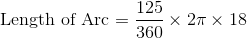Solve.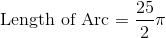### Example Question #94 : Intermediate Geometry

Find the length of an arc if the radius of the circle isand the measurement of the central angle is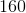degrees.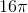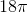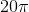Explanation:

An arc is just a piece—or a fraction—of a circle's circumference. Use the following formula to find the length of an arc:Substitute in the given values for the central angle and the radius.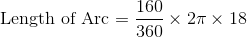Solve.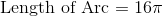### Example Question #95 : Intermediate Geometry

Find the length of an arc if the radius of the circle isand the measurement of the central angle is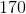degrees.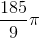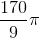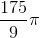Explanation:

An arc is just a piece—or a fraction—of a circle's circumference. Use the following formula to find the length of an arc:Substitute in the given values for the central angle and the radius.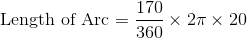Solve.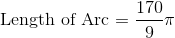### Example Question #96 : Intermediate Geometry

Find the length of an arc if the radius of the circle isand the measurement of the central angle is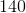degrees.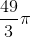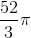Explanation:

An arc is just a piece—or a fraction—of a circle's circumference. Use the following formula to find the length of an arc:Substitute in the given values for the central angle and the radius.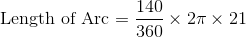Solve.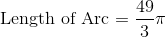### Example Question #97 : Intermediate Geometry

Find the length of an arc if the radius of the circle isand the measurement of the central angle isdegrees.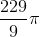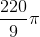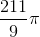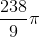Explanation:

An arc is just a piece—or a fraction—of a circle's circumference. Use the following formula to find the length of an arc:Substitute in the given values for the central angle and the radius.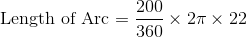Solve.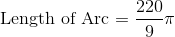### Example Question #98 : Intermediate Geometry

Find the length of an arc if the radius of the circle isand the measurement of the central angle is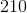degrees.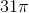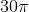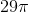Explanation:

An arc is just a piece—or a fraction—of a circle's circumference. Use the following formula to find the length of an arc:Substitute in the given values for the central angle and the radius.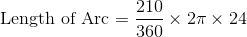Solve.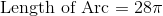### Example Question #99 : Intermediate Geometry

In the figure below,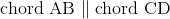. Ifisdegrees, in degrees, what is the measure of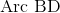?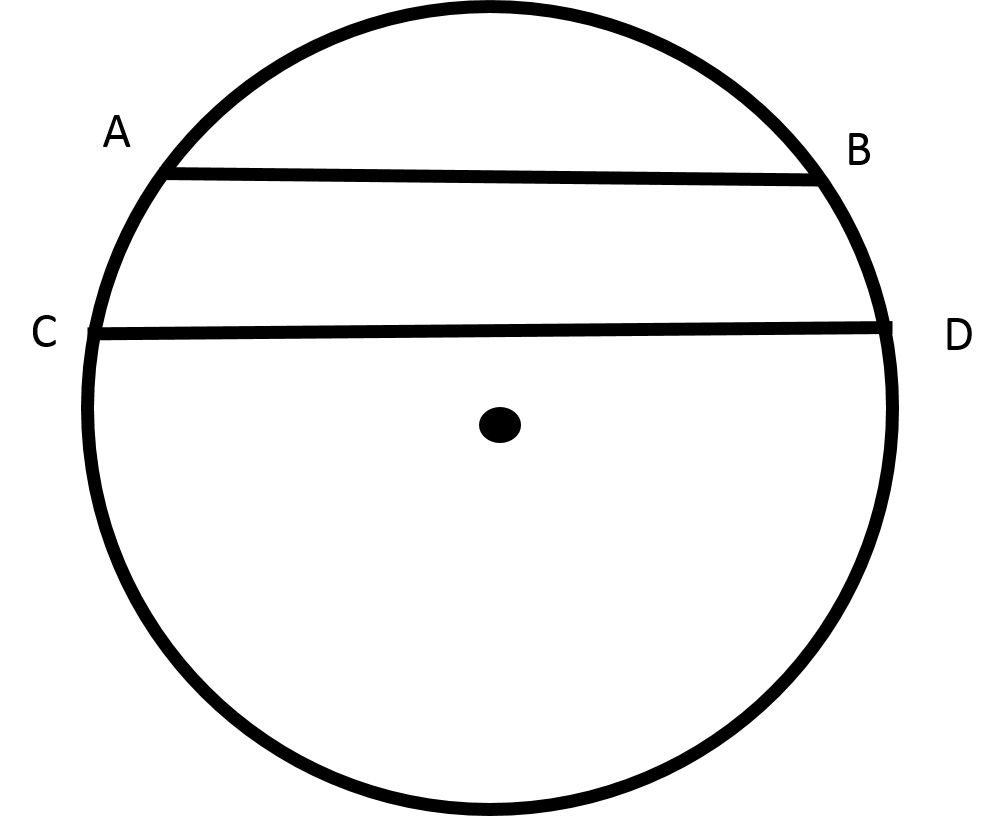The measurement ofcannot be determined with the information given.Recall that when chords are parallel, the arcs that are intercepted are congruent. Thus,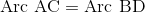.
Then,must also bedegrees.